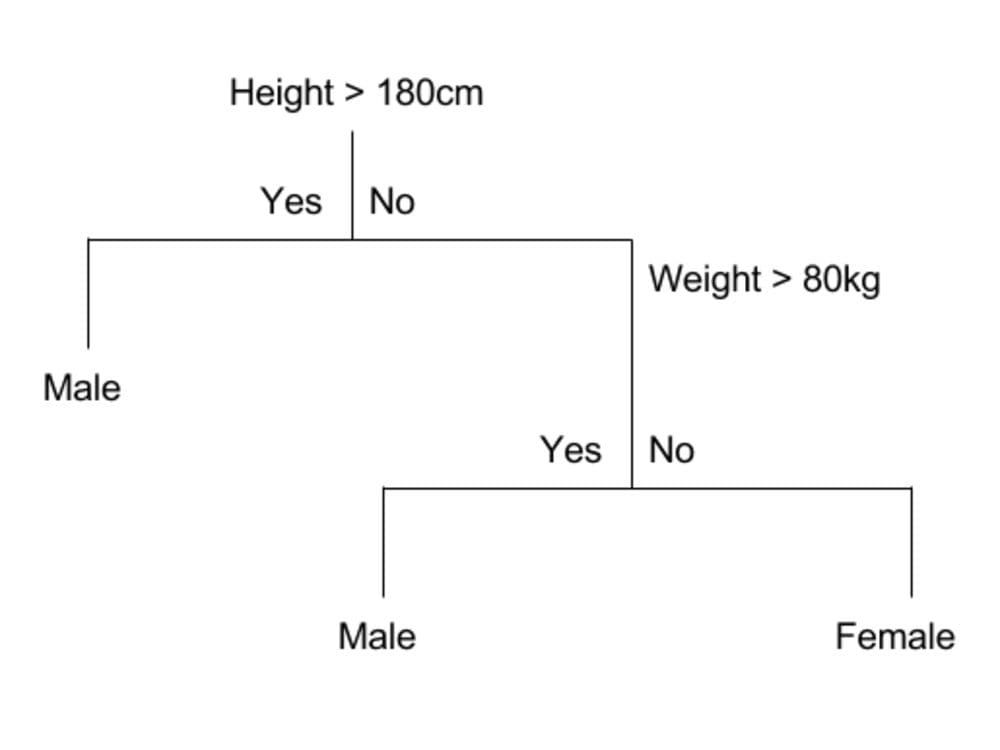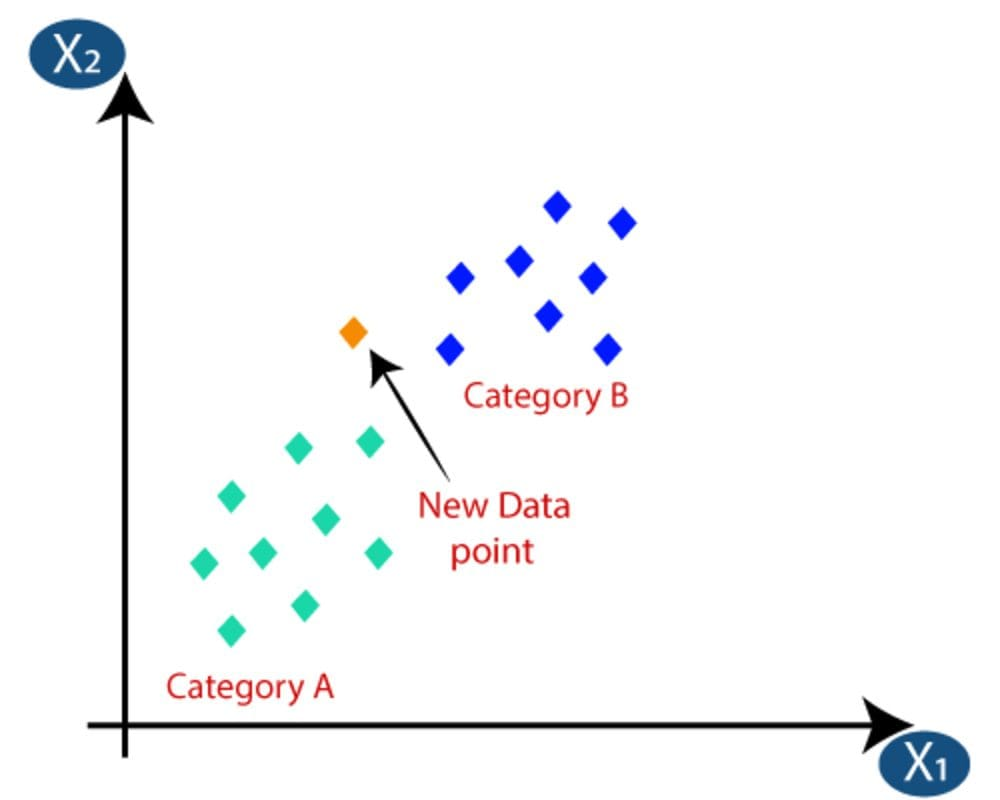# Machine Learning Algorithms for Classification

In this article, we will be going through the algorithms that can be used for classification tasks.Kevin Ku via Unsplash

There are many types of algorithms you can use, so it can be quite overwhelming which one to choose and which one is the right fit for your task.

A good way to distinguish between the different types of algorithms is through their type of learning and the task at hand. I will be going through different types of classification algorithms. But first, let's understand the different types of learning within Machine Learning.

# Types of Machine Learning

There are 3 different types of Machine Learning:

1. Supervised Learning
2. Unsupervised Learning
3. Reinforcement Learning

Supervised Learning is when the algorithm learns on a labeled dataset and analyses the training data. These labeled data sets have inputs and expected outputs.
An example of Supervised Learning algorithms is Logistic Regression.

Unsupervised Learning learns on unlabeled data, inferring more about hidden structures to produce accurate and reliable outputs.
An example of Unsupervised Learning algorithms is K-Means.

Reinforcement Learning is the training of machine learning models to make a sequence of decisions. It focuses on how intelligent agents are to take actions in an environment to maximize the notion of cumulative reward.

# Classification vs Regression:

Supervised learning can be further split into two categories: classification and regression.

Classification is about predicting a label, by identifying which category an object belongs to based on different parameters.

Regression is about predicting a continuous output, by finding the correlations between dependent and independent variables.

Many algorithms can be used for both classification and regression problems. In this article, we will be going through the algorithms that can be used for classification tasks.

## Logistic RegressionSource

Logistic Regression is a Machine Learning algorithm that is used for classification problems and is based on the concept of probability. It is used when the dependent variable (target) is categorical. It is widely used when the classification problem at hand is binary; true or false, yes or no, etc. Logistics regression uses the sigmoid function to return the probability of a label.

For example, it can be used to predict whether an email is a spam (1) or not (0).

## Decision TreeSource

Decision Trees are a non-parametric supervised learning method used for classification and regression. The overall end goal is to build a model that predicts the value of a target variable by learning simple decision rules inferred from the data features.

The concept of Decision trees is in its name. It builds tree branches via a hierarchy approach, where each branch can be considered as an if-else statement. The process of building a Classification Decision tree is through an iterative process of splitting the data into partitions and then splitting it again on each of the nodes. The final classification of the dataset is at the leaves of the decision tree.

For example, classifying the different attributes and characteristics of teenagers pursuing basketball. Your variables of height, weight, ethnicity group will be split into partitions and then split again.

## Random Forests®Source

Random Forests® is a Supervised Learning algorithm and is made up of many decision trees. A good way to remember it is a multitude of trees building up a forest.

The random forest algorithm produces its outcomes based on the predictions generated by the Decision Trees. This prediction is done by taking the average or mean of the output from the various Decision Trees. An increase in the number of trees increases the precision of the outcome. Therefore the higher number of Decision Trees in the forest, the better the accuracy, and overfitting is prevented or at least reduced.

In comparison to Decision Trees, the random forest algorithm model is difficult to interpret and make quick decisions, along with taking a long time.

## K-Nearest Neighbour (KNN)Source

K-nearest neighbors (KNN) algorithm is a supervised machine learning algorithm that can be used to solve both classification and regression problems. The KNN algorithm assumes that similar things exist in close proximity.

KNN uses the idea of similarity, or other words distance, proximity, or closeness. It uses a mathematical approach to calculate the distance between points on a graph. Doing so, it then labels the unobserved data based on the nearest labeled observed data points.

The saying: “Birds of a feather flock together” relates to KNN.

## Support Vector Machine (SVM)Source

Support Vector Machine is a supervised learning model using a linear model that can be used for classification and regression problems.

The concept of the Support Vector Machine algorithm creates a line or a hyperplane which separates the data into classes. It uses the data points that are closer to the hyperplane and influence the position and orientation of the hyperplane; which in turn maximises the margin of the classifier.

RANDOM FORESTS and RANDOMFORESTS are registered marks of Minitab, LLC.

Nisha Arya is a Data Scientist and Freelance Technical Writer. She is particularly interested in providing Data Science career advice or tutorials and theory based knowledge around Data Science. She also wishes to explore the different ways Artificial Intelligence is/can benefit the longevity of human life. A keen learner, seeking to broaden her tech knowledge and writing skills, whilst helping guide others.